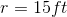# ISEE Upper Level Math : How to find the radius of a sphere

## Example Questions

### Example Question #2 : Spheres

A wooden ball has a surface area of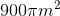.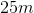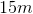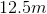Cannot be determined from the information providedExplanation:

A wooden ball has a surface area of.

Begin with the formula for surface area of a sphere: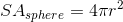Now, plug in our surface area and solve with algebra: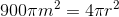Get rid of the pi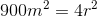Divide by 4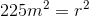Square root both sides to get our answer: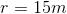### Example Question #3 : Spheres

There is a perfectly spherical weather balloon with a surface area of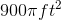, what is its radius?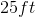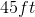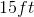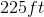Explanation:

There is a perfectly spherical weather balloon with a surface area of, what is its radius?

Begin with the formula for surface area of a sphere:Now, set it equal to the given surface area and solve for r: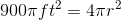First divide both sides by.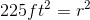Then square root both sides to get our answer: